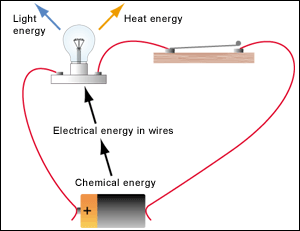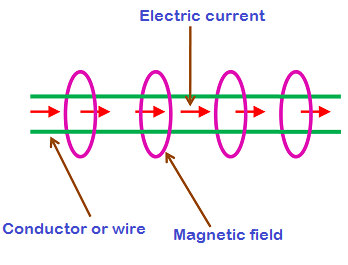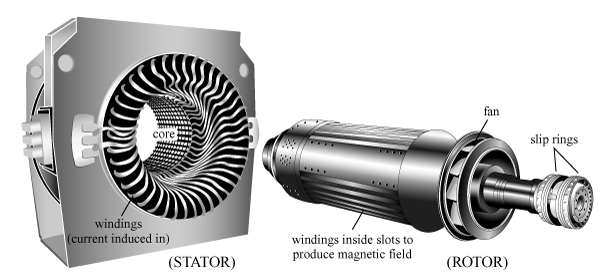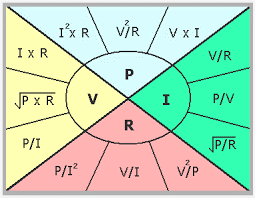×#### Thank you for registering.

One of our academic counsellors will contact you within 1 working day.

Click to Chat

1800-1023-196

+91-120-4616500

CART 0

• 0

MY CART (5)

Use Coupon: CART20 and get 20% off on all online Study Material

ITEM
DETAILS
MRP
DISCOUNT
FINAL PRICE
Total Price: Rs.

There are no items in this cart.
Continue Shopping• Complete Physics Course - Class 11
• OFFERED PRICE: Rs. 2,968
• View Details

```Electrical Energy and Power

Electrical Energy

Production of Electrical Energy

Power

Problems

Summary

Electrical Energy

We know that the law of conservation states that - Energy can neither be created nor be destroyed. It can only be transformed from one form to another. There are mainly two types of energy. They are Kinetic Energy and Potential Energy. The light energy, electrical energy, mechanical energy, all these forms of energy are either kinetic or potential. So electrical energy is one of the many forms of energy.

As the law mentions, we can say that electrical energy can be produced from other forms of energy and also the electrical energy can be transformed to other forms of energy. When an electric bulb is connected to the circuit, chemical energy in cell is converted into electrical energy but not anywhere in the circuit. Then the electrical energy is converted or transformed mainly into light energy and heat energy. In heaters, electrical energy is converted into heat energy. Lightning is the example of electrical energy in nature.Electrical energy conversion

The capacity of doing work is called Energy. In an electric field the energy that is stored in the charged particles is called Electrical Energy.

All things in this universe are made of atoms. Each atom has a nucleus which is surrounded by electrons and the electrons revolve around the atoms. Metals like copper can easily leave their orbits and they are called as Conductors. When the electron escapes from its orbit the electrons moves near the nucleus of another atom. The electric current is produced by the flow of these electrons.

Now consider a magnet. We know a magnet has two poles, a North and South Pole. When two magnets are brought to each other they either attract or repel each other. The magnetic field between the magnets is the one which brings them to move. This magnetic field has an effect on electrons. When a magnet is placed near a conductor like copper, the magnetic field attracts or repels the electrons. This movement of electrons is the electricity.Electricity and magnetism

Production of Electrical Energy

Electricity is generated from the huge generating units that are placed in the hydro electric power stations. The principle of these generators is the same as we can see with the magnet and the copper wire. The rotor and the stator are the two main components of a generator. The rotor consists of a number of magnets. The stator consists of a series of copper bars.

When a magnet is rotated beside the copper bar, the movement of electrons occurs due to the presence of the magnetic field and thus electricity is produced. Likewise the rotor has the same effect as that of the magnet. The rotor is made up of electro magnets. The electro magnets are used when we need a very powerful magnet. It consists of a metal core which is placed inside the coil of electric wire.Rotor and Stator

When the current flows through the electric coil, there occurs a magnetic field. It is able to control the force of magnetism of these electro magnets. As the rotor has many electromagnets they attract and repel the electrons which are present in the copper bars alternatively. Thus the generator generates electricity. It is the mechanical force of water which makes the rotor rotate. When the water hits the blades of the turbine it rotates. This rotating motion of turbine will then drive the shaft which is attached to the rotor. So when the rotor rotates around the stator, the movement of electrons take place.

Consider a circuit with a cell and a resistor. The terminal voltage of the cell is V and the resistance is R. Let us imagine that the total charge of electrons is Q. So the work done to move the charge Q through the potential difference V is the product of the potential energy and the charge which has moved across the potential energy.

W = Q V. We know that the charge Q = current * time = I t.

So W = V I t.

We know that by ohm’s law, Voltage = current * resistance = I R, Substituting in the equation

W = (IR) I t = I2 R t.

Current = Voltage / Resistance = V / R, Thus W = (V / R) R t = (V2 / R) t.

The unit of energy or work is joule.

Electrical energy is the most convenient form of energy as it can be converted to other forms of energy. The heat energy is produced from electrical energy when the electric current flows through the coils of resistive heaters. We have also seen the conversion of electrical energy into light energy by electric bulbs, fluorescent lamps. The electrical energy is converted into mechanical energy in ceiling fans, table fans and other electric motors.

The design and construction of electrical machines are much simpler when compared with the machines of other kinds of energy. So it can controlled easily. For example we can start or stop an electric motor just by putting the switch on or off. The speed of the ceiling fan can be controlled by adjusting the rheostat that is the fan regulator.

The electrical energy can be transported from one place to another by electrical conductors. It can be transported with the speed of the light. As this energy is produced without any fuel cost, the electrical energy is cheaper than any other forms. Solar electric generation system, wind power generation system and hydro electric power generation system plant are different examples for this.

As it does not produce ay poisonous gas or fumes, it makes the nature healthier and clean. So it is used as fuel in machines. It is totally free from pollution to the environment. It can also be transmitted to a very long distance in an efficient way. We have seen that the voltage level can be raised to a desired value. None of the other forms can be transmitted like the electrical energy.

Heating effect and magnetic effect and the effects of electrical current. When we convert electricity to other energy forms some part of energy is converted into heat energy. Joule’s law of heating states that H = I2 Rt. H is the heat produced by an element, I is the current passing through the conductor, R is the resistance offered and t is the time taken. As seen earlier when an electric current is passed through a wire, it also produces a magnetic field around the wire.

Power

Power can be defined as the work done per unit time. Electrical power is the rate of energy consumed in a circuit.

P = W / t = V (Q / t) / t. Here V is the voltage, Q is the charge and t is the time in seconds.

We know that Q / t =I, the current.

So substituting we get P = V I

Consider that the voltage is like the speed at which a car is being driven in a road. The number of cars that passes a mile stone on the road can be compared to the current. So power can be compared as the capacity of the road that can handle the number of cars. The energy consumed can be compared to the number of cars that has driven on the road in an hour.

We know that voltage V = IR.

So W = (IR) I t = I2 R t.

So P = I2 R t / t = I2 R.

We know that I = V / R

Thus W = (V / R) R t = (V2 / R) t.

P = (V2 / R) t / t = V2 / R

The unit of power is joule / second or watt.Power equations

The electric power can be supplied by electric batteries, electric generators etc. Based on electric power, the components of an electric circuit is divided into two. They are passive devices and active devices. Passive devices do not require an external source. They consume electric power within the circuit and then converts into other forms of energy like heat energy, light energy etc. Electric heaters and light bulbs are examples for passive devices. They do not perform function like amplification, oscillation and generation.

In active devices forms of energy like chemical energy, mechanical energy is converted into electric potential energy. Electric generators and batteries are example for this. They produce energy in the form of voltage or current.

Problems

Q1. Find the electric power of an electrical circuit which consumes 100 joules for 10 seconds.

Sol. Energy consumed E = 100 joules

Time t = 10 seconds

So P = E / t = 100 / 10 = 10 watts.

Q2. A circuit has a battery voltage of 30 V. A lamp resistance of 6 Ω is connected to the circuit. Calculate the current and the power of the circuit.

Sol. Current I = Voltage / Resistance = 30 / 6 = 5 A.

Power P = Voltage * Current = 30 * 5 = 150 watts. The lamp gives out 80 W of power in the form of heat and light.

Or Power P = I 2 R = 5 2 * 6 =25 * 6 = 150 watts

Or Power P = V 2 / R = 30 2 / 6 = 900 / 6 = 150 watts.

Q3. A radiator works with a voltage of 20 V and has power of 150 W. Find the resistance and the current flowing through the radiator.

Sol. P = V I

150 = 20 I

I = 150 / 20 = 7. 5 A

R = V / I = 20 / 7.5 = 2. 6 Ω

Summary

The electrical energy can be produced from other different forms of energy. The electrical energy can also be transformed to other forms of energy.

Electricity can be produced by placing a magnet near a conductor like copper. The magnetic field attracts or repels the electrons. With the introduction of magnetic field, the movement of electrons takes place and thus electricity is produced.

W = Q V. Charge Q = current * time = I t. So W = V I t.

W = I2 R t and W = (V2 / R) t.  The unit of energy or work is joule.

Power is the work done per unit time.

P = W / t, P = V I, P = I2 R, P = V2 / R.

The unit of power is joule / second or watt.

Watch this Video for more reference

Electrical Energy and Power
```### Course Features

• 101 Video Lectures
• Revision Notes
• Previous Year Papers
• Mind Map
• Study Planner
• NCERT Solutions
• Discussion Forum
• Test paper with Video Solution